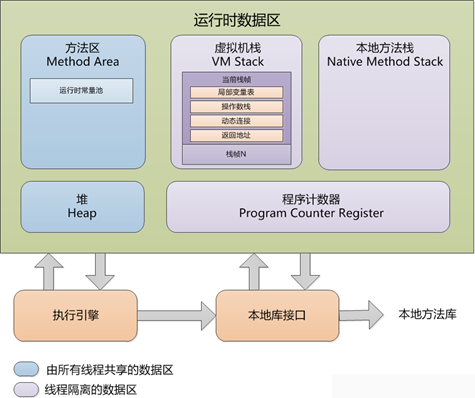[Java 内存]Java内存组成内存数据区域1）程序计数器：在计算机组成结构这门课里面，我们就学到CPU里面有个PC寄存器，这个寄存器主要指CPU当前运行的指令。

2）虚拟机栈（VM Stack）：线程私有，生命周期与线程一样。虚拟机栈描述的是Java 方法执行的内存模型：每个方法被执行的时候都会同时创建一个栈帧（Stack Frame）用于存储局部变量表、操作栈、动态链接、方法出口等信息。栈顶为当前执行的方法。

int a = 3;
int b = 3;

3）本地方法栈（Native Method Stacks），就是native方法咯。
4）方法区（Method Area）线程共享的。主要存放类型信息，包括

• 类的类型，限定符，类名等很多信息
• 变量的变量名，类型，修饰符
• 方法的方法名，参数，返回类型，修饰符
• 类的静态变量
• 常量池（等下会具体说明）

5）堆（Heap），接下来详细介绍一哈Heap。线程共享的。可以动态分配内存大小，生存期也不必事先告诉编译器，因为它是在运行时动态分配内存的。可以参加我的另一篇博客• Eden（Young Generation）：当程序中为一个对象分配内存的时候，这个对象的内存就会被分在这里。在Eden区域进一步被每个线程分割了，也就是说一个线程里面分配的内存都会在一起，而这个区域被称为 Thread Local Allocation Buffer(TLAB)，有了TLAB，避免了昂贵的线程同步问题。当一个TLAB满了之后，内存就会被分配到CommonArea。（线程共享的资源是声明在Common Area里面的，在这里引申出一个问题：多线程对共享资源的访问与控制）。• Survivor（Young Generation）：Survivor会被分为form to，当Eden触发GC之后就会触发Mark-and-Copy算法的GC。把Eden的存活的对象拷贝带Survivor1区域，当Eden再次满了之后，就会把Survivor1与Eden的存活的对象再次拷贝到Survivor2。注意以下几点：每个对象都一个age，每进行一次GC，age就会加一。当age达到一定的值就会被Old Generation区域。或者，Young Generation满了也会copy到Old Generation。（GC的问题可以参见我的其他文章 Java 垃圾回收
//所有的类型信息都会在MethodArea里面进行存储
public class A
{
int a = 0; //局部变量表里面
static int b = 9;//Method Area的静态变量里面
int sayHello(int word)//局部变量表里面
{

}
}

关于String

String这个很容易搞混淆咯。先看代码

• a == b，比较的ab所引用的对象的地址（即在栈里面的地址），而不是内容
• String a = "AAA"，首先检查常量池中有没有"AAA"对象，有的话，返回其引用，没有的话创建一个然后返回一个常量池对象的引用。所以会创建0个或者1个对象
• String b = new String("AAA");首先检查常量池中有没有"AAA"对象，没有的话就创建一个，然后每一次都在堆内存里面也创建一个"AAA"对象，返回一个堆内存的对象的引用。。所以会创建2个或者1个对象
• String一旦确立的，就是不可改变的。而常量池是在编译期间确立的存放在.class文件里面。
可以看到String的构造函数：
• 对于一个String的实例对象a，a.intern()返回的是一个常量池对象的引用
public String(String original) {
this.value = original.value;
this.hash = original.hash;
}

    //String a = "AAA","AAA"对象在常量池咯
//String b = new String("AAA"); "AAA"在堆内存里面
//所以a的地址和b不一样啊
@Test
public void test1() {
String a = "AAA";
String b = new String("AAA");
System.out.println(a == b); // false
}
    //a = "DDD";a指向了"DDD",抛弃了"AAA"
@Test
public void test2() {
String a = "AAA";
String b = "AAA";
System.out.println(a == b); // true
a = "DDD";
System.out.println(a == b); // false
System.out.println(a); // DDD
System.out.println(b); // AAA
}
    //因为1会被转化为String类型的，而且是在编译阶段进行的（因为1已经确定了啊）
@Test
public void test3() {
String a = "AA1";
String b = "AA" + 1;
System.out.println(a == b); // true
}

// i 是变量，在编译阶段不能确定，在运行阶段再能确定，所有不行咯
@Test
public void test4() {
String a = "AA1";
int i = 1;
String b = "AA" + i;
System.out.println(a == b); // false
}

//final会让i在编译阶段确定
@Test
public void test5() {
String a = "AA1";
final int i = 1;
String b = "AA" + i;
System.out.println(a == b); // true
}
    //intern()方法动态扩展常量池
@Test
public void test6() {
String a = "AAA";
String b = new String("AAA");
System.out.println(a == b.intern()); // true  String#intern() 返回的是在常量池里面的地址
}
 @Test
public void test7() {
String fre = "A";
String a = "AA" + fre; //并没有把"AAA"加入到常量池里面
String b = new String("AAA");
String d = "AAA";

//全为false
System.out.println(a == b); //a b 是2个不同的堆对象
System.out.println(a == b.intern()); // 返回的是在常量池里面的地址
System.out.println(a == d); //a指向堆中  d指向常量池
System.out.println(b == d);//b 指向堆中  d指向常量池
System.out.println(d == b.intern()); //true
}

 @Test
public void test8() {
final String fre = "A"; //fianl 使得fre在编译的期间是确定的
String a = "AA" + fre; //将"AAA"添加到了常量池
String b = new String("AAA");
System.out.println(a == b); //a 指向堆中  b指向常量池
System.out.println(a == b.intern()); // b.intern()返回的是在常量池里面的地址
}

关于Integer

    //和String一样的
@Test
public void test() {
Integer a = new Integer(1);
Integer b = new Integer(1);

System.out.println(a == b); //false
}
    // 当基本类型包装类与基本类型值进行==运算时，包装类会自动拆箱。即比较的是基本类型值。
// 具体实现上，是调用了Integer.intValue()方法实现拆箱。
@Test
public void test1() {
int a = 1;
Integer b = 1;
Integer c = new Integer(1);

System.out.println(a == b); //true
System.out.println(a == c); //true
System.out.println(c == b); //false
}

 @Test
public void test1() {
byte a = 1;
Integer b = Integer.valueOf(1);
Integer c = new Integer(1);
System.out.println(a == b.intValue());
System.out.println(a == c.intValue());
System.out.println(c == b);
}

Returns an {@code Integer} instance representing the specified {@code int} value，就是这个咯。

    // Integer a = 1; 会调用这个  Integer a = Integer.valueOf(1);
// Integer已经默认创建了数值【-128-127】的Integer常量池
// 在之间的值就直接在里面取，所以test2可以，test3就不行了
@Test
public void test2() {
Integer a = 127;
Integer b = 127;
System.out.println(a == b); //true

}

@Test
public void test3() {
Integer a = 128;
Integer b = 128;
System.out.println(a == b); //false

}
    // Java的数学计算是在内存栈里操作的
// c1 + c2 会进行拆箱，比较还是基本类型
@Test
public void test4() {
int a = 0;

Integer b1 = 1000;
Integer c1 = new Integer(1000);

Integer b2 = 0;
Integer c2 = new Integer(0);

System.out.println(b1 == b1 + b2); //true
System.out.println(c1 == c1 + c2); //true

System.out.println(b1 == b1 + a); //true
System.out.println(c1 == c1 + a); //true
}

• Interger的常量池只有[-128,127]，所以test中，c == b为false，因为b为常量池对象引用，而c为堆内存对象的引用。
这个在Java8源码里面可以看到：

private static class IntegerCache {
static final int low = -128;
static final int high;
static final Integer cache[];

static {
// high value may be configured by property
int h = 127;
String integerCacheHighPropValue =
sun.misc.VM.getSavedProperty("java.lang.Integer.IntegerCache.high");
if (integerCacheHighPropValue != null) {
try {
int i = parseInt(integerCacheHighPropValue);
i = Math.max(i, 127);
// Maximum array size is Integer.MAX_VALUE
h = Math.min(i, Integer.MAX_VALUE - (-low) -1);
} catch( NumberFormatException nfe) {
// If the property cannot be parsed into an int, ignore it.
}
}
high = h;

cache = new Integer[(high - low) + 1];
int j = low;
for(int k = 0; k < cache.length; k++)
cache[k] = new Integer(j++);

// range [-128, 127] must be interned (JLS7 5.1.7)
assert IntegerCache.high >= 127;
}

private IntegerCache() {}
}
• Integer a = 1; 会调用这个 Integer a = Integer.valueOf(1)即装箱

• intInterger比较：Integer会调用Integer.intvalue()实现拆箱，然后再比较。而IntergerInterger比较，会比较堆内存对象引用或者常量池对象。
• Java的数学计算是在内存栈里操作的

拓展（static与final）

static 的修饰的变量和方法，实际上是指定了这些变量和方法在内存中的”固定位置”－static storage，可以理解为所有实例对象共有的内存空间。static 变量有点类似于 C 中的全局变量的概念；静态表示的是内存的共享，就是它的每一个实例都指向同一个内存地址。把 static 拿来，就是告诉 JVM 它是静态的，它的引用（含间接引用）都是指向同一个位置，在那个地方，你把它改了，它就不会变成原样，你把它清理了，它就不会回来了。 那静态变量与方法是在什么时候初始化的呢？对于两种不同的类属性，static 属性与 instance 属性，初始化的时机是不同的。instance 属性在创建实例的时候初始化，static属性在类加载，也就是第一次用到这个类的时候初始化，对于后来的实例的创建，不再次进行初始化。

final 只对引用的”值”(也即它所指向的那个对象的内存地址)有效，它迫使引用只能指向初始指向的那个对象，改变它的指向会导致编译期错误。至于它所指向的对象的变化，final 是不负责的。